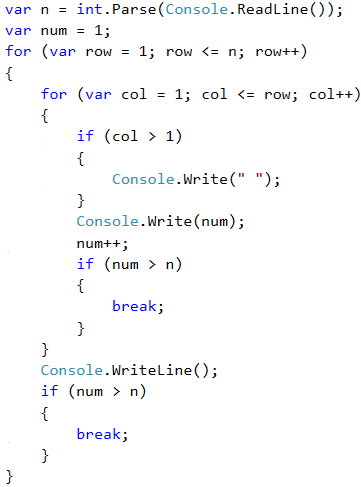# Problem: Numbers Pyramid

Print the numbers 1 … n in a pyramid as in the examples below. On the first line we print one number, on the second line we print two numbers, on the third line we print three numbers, and so on, until the numbers are over. On the last line we print as many numbers as we get until we get to n.

## Sample Input and Output

Input Output Input Output Input Output
7 1
2 3
4 5 6
7
5 1
2 3
4 5
10 1
2 3
4 5 6
7 8 9 10

## Video: Pyramid of Numbers

Watch this video lesson to learn how to draw a pyramid of numbers using nested loops and the break operator: https://youtu.be/SWU-gQa31QI.

## Hints and Guidelines

We can solve the problem with two nested loops (by rows and columns) with printing in them and leaving when the last number is reached. Here is the idea, written in more details:

• We create a variable n, to which we assign an integer value from the console input.
• We create a variable num with an initial value of 1. It will keep the number of printed numbers. At each iteration we will increase it by 1 and print it.
• We create an outer for loop that will be responsible for the rows in the table. We name the variable of the loop row and set an initial value of 0. For condition, we set row < n. The size of the step is 1.
• In the body of the loop we create an inner for loop that will be responsible for the columns in the table. We name the variable of the loop col and set an initial value of 0. For a condition, we set col < row (row = number of digits per line). The size of the step is 1.
• In the body of the nested loop:
• We check if col > 1, if yes –> we print space. If we do not do this, but directly print the space, we will have an unnecessary one at the beginning of each line.
• We print the number num in the current cell of the table and increase it by 1.
• We are checking for num > n. If num is greater than n, we interrupt the running of the inner loop.
• We print a blank line to move to the next one.
• Again, we check if num > n. If it is greater, we interrupt our program by break.

## Implementation of the Idea

Here is an example implementation: